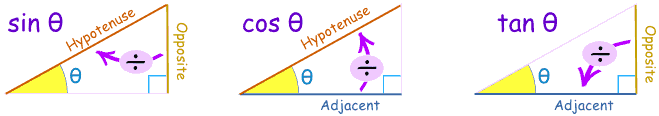So how do you move things around a 2D screen? I covered this early on as part of introducing gravity, but I haven’t written about it on its own – until now.

I’m going to assume we have something we want to move around the screen, under the control of either the program or the user. I’ll assume the user, to make it more interesting, and to cover the touch code needed to manage it. It involves simple trigonometry, but nothing nasty, I promise.

Separate x,y controls

The simplest approach (for coding) I can think of is this. If you click in the

• top (or bottom) 1/4 of the screen, x axis speed increases (or reduces)
• left (or right) 1/4 of the screen, y axis speed increases (or reduces)
• if you click in the middle of the screen, you stop.
• .
Here is the touch code, where dx and dy are the speed along the x and y axes, in pixels per second:

function touched(touch)
local t=false --to tell us if touch has been located yet
--change y speed if in outer 1/4 of screen
if touch.yHEIGHT*.75 then dy=dy+s  t=true
end
--change x speed if in top/bottom 1/4 of screen
if touch.xWIDTH*.75 then dx=dx+s   t=true
end
--stop moving otherwise
if t==false then dx,dy=0,0 end
end

You can try this yourself here

You will see, however, it’s a bit difficult to manage your direction accurately. When I was creating my 3D town, it was very important to be able to turn smoothly to look around, so I changed the approach above so that if you touch the left or right of the screen, you turn left or right (ie change your angle) rather than affecting your x axis position.

This means that if you want to go somewhere, you turn in that direction and go forwards (or backwards). Which is pretty much like real life.

Now the code gets pretty interesting. We have an angle, and a speed, and we have to turn that into a change in x and y values. We can calculate them using simple trigonometry, with the help of these diagramsLook at the left hand one (sin) first. We know the angle Θ, and the speed, which is the hypotenuse. We want to calculate the other two sides – the “opposite” side is our y, and the “adjacent” side is our x.

Now sin(Θ) = opposite / hypotenuse = y value/ speed

So therefore y value = speed * sin(Θ)

Similarly, cos(Θ) = adjacent / hypotenuse = x value / speed

so x value is speed * cos(Θ)

These give us the change in x and y, which we add to the existing values.

If you try the code here, you can navigate around the screen. I’ve used an ellipse so you can see which way you are pointing. Remember that you always go forward in the direction you are pointing, so if that is down the screen, you will move down, not up.

Drawing objects at an angle

There is a little – but important – trick hidden in the code. To draw my ellipse at an angle, I need to rotate the screen first (in the same way you turn a piece of paper round if you have to draw something at an odd angle). The sequence of steps is

• store screen setup with pushMatrix()
• move the drawing origin, 0,0, to x,y, where you want to draw the ellipse
• rotate the screen by the negative of the angle
• draw the ellipse
• restore the original screen setup with popMatrix()
• .
The negative of the angle? Huh?

Let’s think about a real life example. Suppose you have to draw something on a big sheet of paper, nearly upside down, over in a corner of the paper. The obvious thing to do is pull the paper toward you so the place you want to draw is neatly under your pencil (ie translate), turn the paper round so you can draw the image the right way up (ie rotate), and when you’re done, you put the paper back the way it was (ie popMatrix).

Now let’s use this example to focus on the angle question. Suppose you want to draw the image on its right side, so if it was a person, the feet will be on the left and the head on the right. You turn the paper to the left, and draw the image normally, then turn the paper back to the right, so the image now has its feet to the left. Can you visualise that? It may help if you try it for real.

Another way to see this is that the screen is turned twice – before drawing the image, and after drawing the image (when the original turn is reversed). The image is not affected by the first turn, but only by the second turn. So if we want the image turned by +30 degrees, our first turn needs to be -30 degrees, so the image will turn by +30 degrees when the first turn is reversed.

Despite all these explanations, it still catches me out every time (sigh).

Moving smoothly

I’ve posted about this recently, but it’s worth repeating. If your program gets complex, you may find your objects moving in slow motion. You can ensure the speed stays at the correct pixels per second by multiplying it by DeltaTime.

DeltaTime is the time since last redraw, so if you multiply it by pixel speed/second, you get the correct distance to move, however fast your program is running. (That’s why I usually express speed in pixels per second, rather than pixels pre redraw).